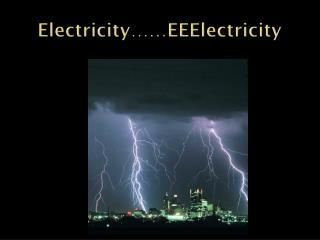DownloadDownload PresentationElectricity…… EEElectricity

# Electricity…… EEElectricity

Télécharger la présentation## Electricity…… EEElectricity

- - - - - - - - - - - - - - - - - - - - - - - - - - - E N D - - - - - - - - - - - - - - - - - - - - - - - - - - -
##### Presentation Transcript

1. Electricity……EEElectricity

2. Electricity Chp 20 • Electrostatics, the study of electric charges • The evidence of electricity has been noted since 2700 BC • Lets review some basics: • Like charges repel • Opposite charges attract • An insulator is an object that a charge or energy can not move easily through • A conductor is an object that a charge or energy can move easily through.

3. More Basics • The Atom • Composed of Protons (+), Electrons (-) and Neutrons • Protons are found in the nucleus, along with Neutrons. Electrons orbit the nucleus. • In a neutral atom, the number of electrons equals the number of protons • Electric charges exert a force on other charges at a distance • This force is stronger when the charges are closer together

4. Even More Basic Stuff • The charge possessed by a single electron and proton are equal at 1.60 x 10-19 C. • The magnitude of the charge on an electron is called the elementary charge • C = 1 Coulomb the SI unit for charge • 1 Coulomb of charge is equal to 6.24 x 1018 electrons. • A typical lightning bolt has 5 – 25 C • A nickel can have up to 106 C of charge balanced by positively charge protons.

5. Static Electricity

6. More Static Eelctricity

7. How? • Typically two non conductive or neutral objects in contact with each other will result in the transfer of electrons. • Air is non conductive but lightning and sparks fly across it. If the unbalance of electrons or protons is large enough, almost anything can become a conductor. In the case of air electrons are forced to move across it creating a plasma of charged atoms and freely moving electrons we see as lightning.

8. More charges • Electric charges can be move through induction or conduction. • Induced charges are charges brought on without physical contact. If a source of electrons or negative charge is brought next to two objects in contact the electrons will move from one to the other creating a positively charged object and a negatively charged one

9. More • Charging by conduction is where charges are brought by physical contact. A source of negative charge brought in contact with another object will transfer those excess electrons. • Charges will separate based on their polarity. • Electrostatic forces can be useful and destructive.

10. Charges?! We don’t need no stinking Charges. • As stated earlier. Force depends on distance and is the inverse square of the distance • F = 1/r2 • Force depends on charge F = qaqb where q represents charge and (a) and (b) are charge if two objects

11. Coulombs Law • Coulomb was a French physicist around 1785 and established these relationships which are summarized in the following equation: • F = K qaqb/ r2 • Which states the force (F) between two objects (q) is equal to coulombs constant (K) times the product of the two charges (q) in Coulombs, divided by the square of the distance between them (r) in meters • K = 9.0 x 109 N – m2/C2 Remember this electric forces are vector quantities with magnitude and direction, sorry, more trig.

12. Lastly • We will play with Coulombs law tomorrow and the next day and the next day……